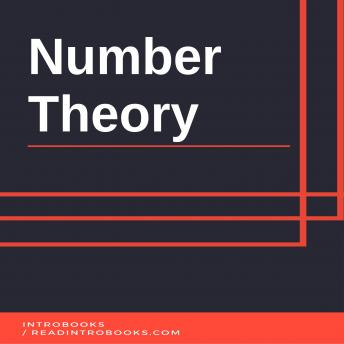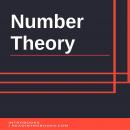# Number Theory

#### Summary:

In old times, number theory was also known as arithmetic. However, now arithmetic and number theory are considered as separate branches from each other's, it was not same in old times. Number theory is one of the many important branches of pure mathematics. This branch is mainly dedicated and includes study about integers. This theory describes many fundamental and basic concepts of mathematics that were used to develop modern concepts. Thus, number theory is often referred as "Queen of Mathematics". In number theory, following concepts are described:
Concept of prime numbersProperties of objects that are derived through integersGeneralization of integersRational numbers and algebraic integers are significant concepts that are included in number theory. In number theory, integers are considered as a solution to a particular problem. This concept is known as Riemann Zeta Function. However, it is not necessary to consider them as solution only; they can also be considered in themselves. Study of analytical objects helps to understand questions in number theory. Properties of integers, prime numbers and number-theoretic objects are described in Riemann Zeta Function. These properties can be studied descriptively in a separated branch named analytic number theory. In Diophantine approximation, real numbers are learnt in ration to relational number.
In older terms, arithmetic was used to refer number theory. However, it was separated in early 20th century. Arithmetic word is now used to refer to general elementary calculations. Term arithmetic is now used in many fields such as:
Mathematical logicPeano arithmetic Computer scienceFloating point arithmeticIn late 20th century, French theorists leaved a noticeable impact on number theory. Due to their influence, they again related tern arithmetic with number theory. However, many theorists argued upon this and denied to accept this as it was already proven false in past time. However, term arithmetical is now considered as adjective to number-theoretic. Early 20th century was a golden time for development of number theory, especially the time span of 1930s and 1940s. Many important results were acquired in that period. Later on, 1970s was proven an important period as well with the development of computational complexity theory.
Number theory is an important branch of pure mathematics since it contains many basic concepts that are used to build up complex concepts of pure mathematics. One who is looking for a breakthrough in broad term mathematics is suggested to start from this theory. It will clear up basic concepts so it will be surprisingly easy to understand complex concepts. This short book describes all the basic concepts without going in too deep. So, one can use this basic knowledge to understand complex concepts easily and effectively.

## Number Theory

#### by Introbooks##### Enter your email below to be notified as soon as it is available!

By clicking "Notify Me" you consent to receiving electronic marketing communications from Audiobooks.com. You will be able to unsubscribe at any time.